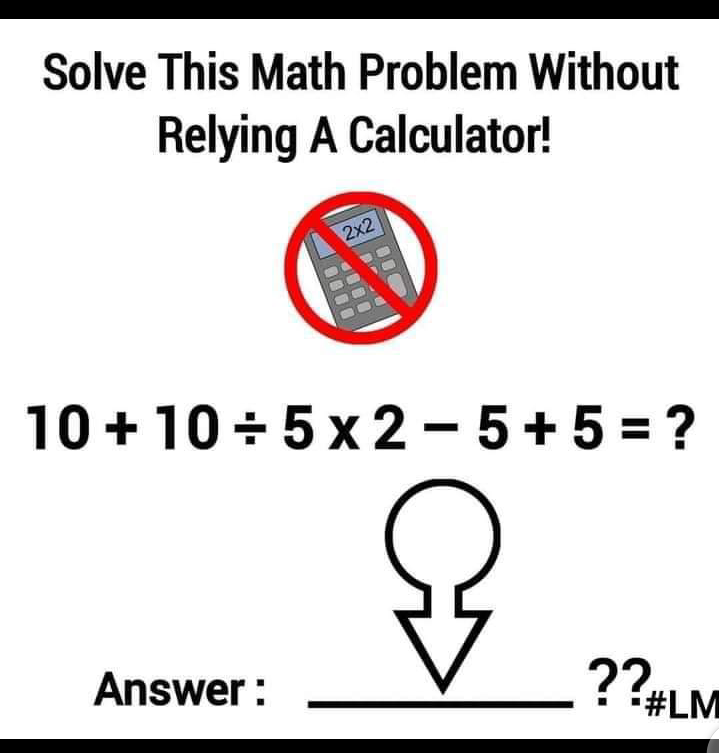0

# What's 10+ 10 ÷ 5×2-5+5=? answerThis math quiz '10+ 10 ÷ 5×2-5+5=?' has gone viral on social media, many people  who tried to solve it have answers like 4, 10 , 8, 14 etc.

If you have been doubting your answer, then in this article I will solve and explain  in detail why the answer to 'What's 10+ 10 ÷ 5×2-5+5=?' Math Quiz is not  8. Be sure to share the Quiz to those who love Quizzes, puzzles, riddles and brain games.

I will solve Taking into consideration some Basic Mathematical rules such as :

• Order of Operation (BODMAS and PEMDAS).

• Solving from left to right.

• Signs lie behind a number. This rule is affecting many answers so you have to take it seriously and master your number line well.

## Solution: What's 10+ 10 ÷ 5×2-5+5=?

The correct answer to this tricky math quiz would be 14 not 4. Full solving and explanation below.

The solution to this question is simple but tricky. Many people always fall into the trap of not using basic mathematical principles and rules such as BODMAS and PEMDAS

BODMAS stands for:

B=Bracket,

O=Order of operation,

D=Division,

M=Multiplication,

S=Subtraction

PEMDAS stands for:

P=Parentheses,

E=Exponents,

M=Multiplication,

S=Subtraction.

So , using BODMAS,

10+ 10 ÷ 5×2-5+5=?

First Division: 10÷ 5 = 2.

Secondly, multiply: 2 × 2 = =4.

The Quiz will Now be 10 + 4 -5+5 = ?

Thirdly, addition 10+4 + 5 = 19.

Quiz will now be 19-5=?

Lastly, substraction,

So, 19 - 5 = ?

14 = ?

Summary answer to 10+ 10 ÷ 5×2-5+5=? Math Quiz

10+10÷5×2-5+5

10+2×2-5+5

10+4-5+5

10+4

14 ans BODMAS

Therefore, The answer to 5+5×5 +5 is 14, use a number line or a calculator to confirm your answer.